Open in App
Not now

# Aggregating and analyzing data with dplyr | R Language

• Last Updated : 21 Oct, 2022

The dplyr package is used in R language to perform simulations in the data by performing manipulations and transformations. It can be installed into the working space using the following command :

`install.packages("dplyr")`

There are a large number of inbuilt methods in the dplyr package that can be used in aggregating and analyzing data. Some of them these methods are as follows :

## Using Filter Method

The filter method in the dplyr package in R is used to select a subset of rows of the original data frame based on whether the specified condition holds true. The condition may use any logical or comparative operator to filter the necessary values.

Syntax : filter(data , cond)

Arguments:

data- the data frame to be manipulated

cond-  the condition to be checked to filter the values

In the following code snippet, we are checking for all the rows that correspond to marks in the subject maths. All the rows are returned wherein the students have marks corresponding to the subject maths. Two such rows are returned from the original database.

## R

 `#installing the required libraries` `library``(dplyr)` `#creating a data frame` `data_frame = ``data.frame``(subject = ``c``(``"Maths"``,``"Hindi"``,``"English"``,``"English"``,``"Hindi"``,` `                                   ``"Maths"``,``"Hindi"``),` `                        ``marks = ``c``(34,23,41,11,35,67,87))` `print``(``"Original Data frame"``)` `print``(data_frame)` `print``(``"Data frame with maths subject"``)` `filter``(data_frame, subject == ``"Maths"``)`

Output :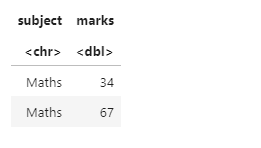## Mutate Method

The Mutate method in the dplyr package is used to add modify or delete the original data frame columns. A new column can be added by specifying the new column name and the formula used to compute the value within this column.

Syntax : Mutate(new-col-name=formula)

Arguments:

new-col-name- the name of the new column to be added

formula-the formula to compute the value of the newly added column.

In the following code snippet, a new column named new_marks is added to the data frame wherein 10 marks is added as grace marks to the existing marks of the various students

## R

 `#installing the required libraries` `library``(dplyr)` `#creating a data frame` `data_frame = ``data.frame``(subject = ``c``(``"Maths"``,``"Hindi"``,``"English"``,``"English"``,``"Hindi"``,` `                                   ``"Maths"``,``"Hindi"``),` `                        ``marks = ``c``(34,23,41,11,35,67,87))` `print``(``"Original Data frame"``)` `print``(data_frame)` `print``(``"Data frame with 10 grace marks added to all marks"``)` `#adding 10 grace marks to all marks` `data_frame %>%` `  ``mutate``(new_marks = marks+10)`

Output :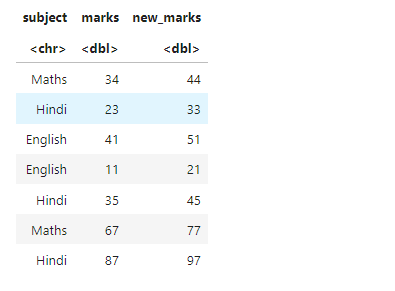## Select Method

The Select method in the dplyr package is used to select the specified columns from the data frame. The columns are retrieved in the order in which they occur in the definition of this method all the rows are retained for these columns.

Syntax : select(list-of-columns-to-be-retrieved)

In the following code snippet, the columns name and marks are extracted from the database in the order such that the name column appear before the marks column

## R

 `#installing the required libraries` `library``(dplyr)` `#creating a data frame` `data_frame = ``data.frame``(subject = ``c``(``"Maths"``,``"Hindi"``,``"English"``,``"English"``,``"Hindi"``,` `                                   ``"Maths"``,``"Hindi"``),` `                        ``marks = ``c``(34,23,41,11,35,67,87),` `                        ``name = ``c``(``"A"``,``"V"``,``"B"``,``"D"``,``"S"``,``"Y"``,``"M"``))` `print``(``"Original Data frame"``)` `print``(data_frame)` `print``(``"Data frame with 10 grace marks added to all marks"``)` `#selecting name and marks from the data frame` `data_frame %>%` `  ``select``(name,marks)`

Output :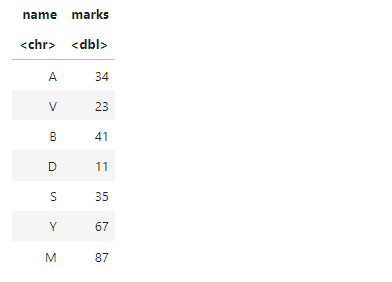## Using Group_by and Summarise

The group_by method is used to divide the data that is available in the data frame into segments based on the groups that can be created from the specified column name. The group_by method may contain one or more columns.

Syntax : group_by(list-of-columns-to-used-for-grouping)

In the following code snippet, the subject column has been used to group the data.

Now this data frame is subjected to the summarise operation wherein the new column can be created by using available inbuilt functions to calculate the number of entries falling in each group summarise(new-col-name=n()).

The n() method is used to return the counter of values following in each group.

In the following code snippet, for instance the number of students studying English were 2 so 2 is displayed for the subject english.

## R

 `#installing the required libraries` `library``(dplyr)` `#creating a data frame` `data_frame = ``data.frame``(subject = ``c``(``"Maths"``,``"Hindi"``,``"English"``,``"English"``,``"Hindi"``,` `                                   ``"Maths"``,``"Hindi"``),` `                        ``marks = ``c``(34,23,41,11,35,67,87),` `                        ``name = ``c``(``"A"``,``"V"``,``"B"``,``"D"``,``"S"``,``"Y"``,``"M"``))` `print``(``"Original Data frame"``)` `print``(data_frame)` `print``(``"Calculating students in each subject"``)` `#grouping the data frame by subject` `data_frame %>%` `  ``group_by``(subject) %>%` `  ``summarise``(sum_marks = ``n``())`

Output :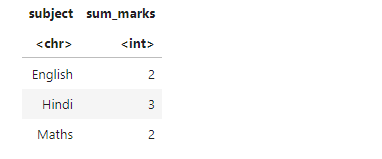## Using Aggregate Functions

Instead of the inbuilt methods aggregate methods like sum() or mean() can be used to provide statistical information for the data. For instance, in the summarise method we have used the summarise function with the sum method taking in argument as marks the sum of the marks falling in each category of the subject are then displayed as the output.

## R

 `#installing the required libraries` `library``(dplyr)` `#creating a data frame` `data_frame = ``data.frame``(subject = ``c``(``"Maths"``,``"Hindi"``,``"English"``,``"English"``,``"Hindi"``,` `                                   ``"Maths"``,``"Hindi"``),` `                        ``marks = ``c``(34,23,41,11,35,67,87),` `                        ``name = ``c``(``"A"``,``"V"``,``"B"``,``"D"``,``"S"``,``"Y"``,``"M"``))` `print``(``"Original Data frame"``)` `print``(data_frame)` `print``(``"Calculating sum of marks of students in each subject"``)` `#grouping the data frame by subject` `data_frame %>%` `  ``group_by``(subject) %>%` `  ``summarise``(sum_marks = ``sum``(marks))`

Output :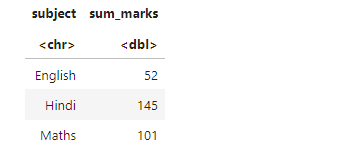My Personal Notes arrow_drop_up
Related Articles